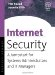# List of Figures

Figure 1.1
Figure 1.2
Figure 1.3
Figure 1.4
Figure 1.5
Figure 1.6
Figure 1.7
Figure 1.8
Figure 1.9
Figure 1.10
Figure 1.11
Figure 1.12
Figure 1.13
Figure 1.14
Figure 1.15
Figure 1.16
Figure 1.17
Figure 1.18
Figure 1.19

Figure 2.1
Figure 2.2
Figure 2.3

Figure 3.1
Figure 3.2
Figure 3.3
Figure 3.4
Figure 3.5
Figure 3.6
Figure 3.7
Figure 3.8
Figure 3.9
Figure 3.10
Figure 3.11

Figure 4.1
Figure 4.2
Figure 4.3
Figure 4.4
Figure 4.5
Figure 4.6
Figure 4.7
Figure 4.8

## Chapter 5: Protecting Your Intranet From the Extranet and Internet

Figure 5.1
Figure 5.2
Figure 5.3: SSL key generation time in milliseconds.
Figure 5.4
Figure 5.5

Figure 6.1
Figure 6.2

Figure 7.1
Figure 7.2
Figure 7.3
Figure 7.4
Figure 7.5
Figure 7.6
Figure 7.7
Figure 7.8

Figure 8.1
Figure 8.2
Figure 8.3
Figure 8.4
Figure 8.5

## Chapter 10: Disaster Recovery

Figure 10.1
Figure 10.2
Figure 10.3Internet Security: A Jumpstart for Systems Administrators and IT Managers
ISBN: 1555582982
EAN: 2147483647
Year: 2003
Pages: 103
Authors: Tim Speed, Juanita Ellis